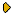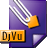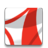Журнал Экспериментальной и Теоретической Физики
 НАЧАЛО | ПОИСК | ДЛЯ АВТОРОВ | ПОМОЩЬОбщая информация о журналеЗолотые страницыАдреса редакцииСодержание журналаСообщения редакцииПравила для авторовЗагрузить статьюПроверить статус статьиЖЭТФ, Том 146, Вып. 2, стр. 313 (Август 2014)
(Английский перевод - JETP, Vol. 119, No 2, p. 372, August 2014 доступен on-line на www.springer.com )

STATISTICAL THEORY OF DIFFUSION IN CONCENTRATED ALLOYS

Поступила в редакцию: 20 Января 2014

DOI: 10.7868/S0044451014080100DJVU (356.9K)PDF (464.7K)

The earlier-suggested master equation approach is used to develop the statistical theory of steady-state diffusion in concentrated substitution alloys considering FCC alloys with the nearest-neighbor pairwise interactions as an example. General expressions for the Onsager coefficients in terms of microscopic interatomic interactions and some statistical averages are presented. We discuss methods of calculations of these averages using various statistical approximations and various approximations for description of vacancy correlations, with the full taking into account the vacancy-solute interactions. Our simplest statistical approximation, called the ``kinetic mean-field approximation'' (KMFA), corresponds to using the mean-field approximation for statistical averages and the pair-cluster approximation (PCA) for calculations of thermodynamic parameters; for dilute alloys, the KMFA is exact. To describe vacancy correlation effects at any concentrations, we develop both the nearest-neighbor-jump approximation and the second-shell-jump approximation. We also describe methods to take into account fluctuations in statistical averages using the PCA, and to describe non-pairwise vacancy-solute correlations using the triple vacancy-solute correlation model. For each of approximations and methods developed, we derive expressions for the Onsager coefficients at any composition of an alloy. For binary alloys, we also present expressions for the diffusion coefficients. The results obtained can provide a basis for microscopic calculations of diffusion coefficients at any composition of an alloy.

Сообщить о технических проблемах